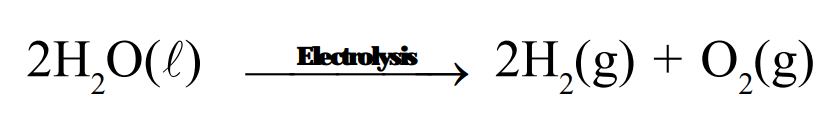# Why are decomposition reactions called the opposite of combination reactions ?Question.
Why are decomposition reactions called the opposite of combination reactions ? Write equations for these reactions.

solution:
Decomposition reactions are those in which a compound breaks down to form two or more substances. These reactions require a source of energy to proceed. Thus, they are the exact opposite of combination reactions in which two or more substances combine to give a new substance with the release of energy.

$\mathrm{AB}+$ Energy $\longrightarrow \mathrm{A}+\mathrm{B}$Combination reaction

$\mathrm{A}+\mathrm{B} \longrightarrow \mathrm{AB}+$ Energy

$2 \mathrm{H}_{2}(\mathrm{~g})+\mathrm{O}_{2}(\mathrm{~g}) \longrightarrow 2 \mathrm{H}_{2} \mathrm{O}(\ell)+$ Energy